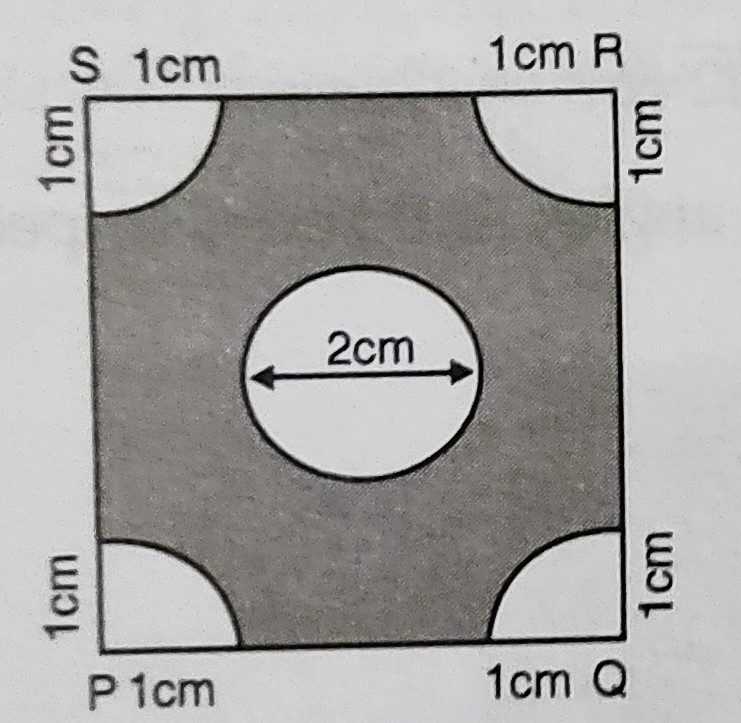# In figure below, $P Q R S$ is a square of side $4 \mathrm{~cm}$. Find the area of the shaded square."

Given:

$P Q R S$ is a square of side $4 \mathrm{~cm}$.

To do:

We have to find the area of the shaded square.

Solution:

Let the side of the square $PQRS$ be $a$.

This implies,

$a= 4\ cm$

Area of the square $PQRS= a^2$

$= 4^2=16\ cm^2$

Radius of each of the four quadrants at the corners $r= 1\ cm$

Area of 4 quadrants $=4 \times \frac{1}{4} \pi r^{2}$

$=\pi r^{2}$

$=\pi \times 1^2$

$=\pi \mathrm{cm}^{2}$

Radius of the inner circle $=\frac{2}{2}$

$=1 \mathrm{~cm}$

This implies,

Area of the inner circle $=\pi r^{2}$

$=\pi \times 1^2$

$=\pi \mathrm{cm}^{2}$

Therefore,

Area of the shaded region of the square $=16-\pi-\pi$

$=(16-2 \pi) \mathrm{cm}^{2}$

The area of the shaded square is $(16-2 \pi) \mathrm{cm}^{2}$.